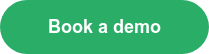Compare Kreo Takeoff and Revit’s QTO tool before your quantity takeoff

According to a survey Kreo ran with Construction Manager Magazine, quantity takeoff (QTO) is the top process in cost estimation people would like to automate. The survey included quantity surveyors, cost estimators and project managers, among other architecture, engineering and construction professionals.

This is a crucial aspect of the pre-construction planning process to get right, as it impacts both the cost and schedule of any project. We compared Kreo's brand new QTO tool to Revit's more established tool.

Kreo's Quantity Takeoff Tool

What differentiates Kreo Takeoff from other QTO tools in general is its geometry library. It measures Building Information Modeling (BIM) quantities according to the element’s meshes. To clarify, that means it doesn’t read any other element properties to get quantities. When the library calculates certain quantities, e.g. length, it measures length of the guiding line of the element. See the example in Diagram 1 below:

Diagram 1In Diagram 1, above, Kreo calculates the column length to be 3100 mm. When we compare this with a similar test in Autodesk Revit, the length of the same column is 3400 mm (see Diagram 1a below).

Diagram 1aKreo lets you check Revit properties directly within the quantity takeoff page (see Diagram 1b).

Diagram 1bWhich calculation is right, Kreo’s or Revit’s? Let’s find out. The column volume given in the Revit properties is 0.496 m3. But when we calculate the volume depending on the Revit dimensions we get the following:

V = b x h x l = 0.4 x 0.4 x 3.4 = 0.544 m3

So, the volume depending Revit dimensions is not equal to the volume from Revit properties. Let’s check the properties from Kreo Takeoff:

l = V/ (b x h) =0.496/(0.4 x 0.4) =3.1 m

Why is the Revit length wrong?

Designers always create columns firstly then floors. When they create floors they use the join function from Revit which solves intersections between columns and floors by decreasing column length. But the column length in properties isn’t updated.

How can this affect project cost?

Incorrect quantities will increase total project cost for its impact on many activities, including (but not limited to):

• Labour cost because of level conditions (length)
• Labour productivity because of level conditions (length)
• Column formwork (side area and length for concrete forms)
• Concrete curing (side area)
• Concrete finishing (side area)
• Fine finish (side area)

Diagram 2Kreo calculated that beam cross-section dimensions (b x h) are 200x450 mm and the length is 1900 mm (see Diagram 2 above). But the properties from Revit give other values: 400x450 mm for cross-section and 2000 mm for length. If we get close to the beam on the 3D view (see Diagram 2a) we can see how Kreo’s calculation mirrors reality.

Diagram 2aLooking into the calculations more closely:

V = b x h x l = 0.4 x 0.45 x 2 = 0.36 m3

But in Revit properties, we find that the volume is 0.171 m3.

If we look into the Kreo quantities, this is what find:

V = b x h x l = 0.2 x 0.45 x 1.9 = 1.71 m3

So we get the same volume as the volume from properties. This example shows that three basic dimensions from Revit are wrong.

Why is the Revit length wrong?

For exactly the same reason as we saw in the first example, Diagram 1.

How can it affect project cost?

Incorrect quantities increase total project cost their impact on many activities, including (but not limited to):

• Beam formwork (side and bottom area; length for concrete forms)
• Concrete curing (side and bottom area)
• Concrete finishing (side and bottom area)
• Fine finish (side and bottom area)

In our final analysis, we looked at cross-section dimensions. Kreo calculated that column cross-section dimensions were 316 x 316 mm (look at the picture above). But the properties from Revit gave other values: 400x400 mm.

Diagram 3This is what we see when we look into Revit quantities:

V = b x h x l = 0.4 x 0.4 x 1,61 = 0.2576 m3

But in Revit properties, we can find that the volume is 0.161 m3. Then when we look at the quantities in Kreo Takeoff, we get:

V = b x h x l = 0.316 x 0.316 x 1.61 = 0.1607 m3

This is almost the same volume as the volume from properties.

This example shows that cross-section dimensions from Revit are wrong.

Why is the length from Revit wrong?

For the same reasons as our first example, Diagram 1.

How can this affect project cost?

Incorrect quantities increase the total project cost for their impact on many activities, including:

• Column formwork (side area and length for concrete forms)
• Concrete curing (side area)
• Concrete finishing (side area)
• Fine finish (side area)

Book a demo with the Kreo Team to see how our product works in detail. You'll get more accurate quantity takeoff than ever before in record time.